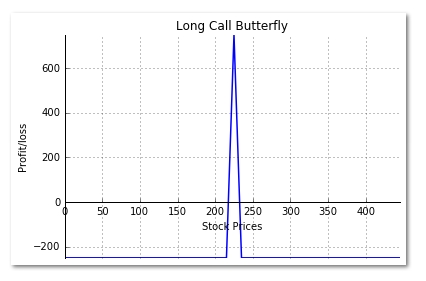# Long Call Butterfly Strategy on Python

We talked about Covered Call Strategy in a previous article. In this post, we will cover the Long Call Butterfly. The Long Call Butterfly is a popular strategy deployed by traders when little price movement is expected in the underlying security. The Long Call Butterfly strategy involves three legs:

• Buying a lower strike In-the-money (ITM) Call option
• Buying a higher strike Out-of-the-money (OTM) Call option
• Selling two At-the-money (ATM) Call options

In this strategy, all Call options have the same expiration date, and the distance between each strike price of the constituent legs must be the same. Let us take an example to understand the working of a Long Call Butterfly, its payoff, and the risk involved in the strategy.

#### Example:

ABC stock is trading at Rs. 225 on Jan 2nd, 2015. To create a Long Call Butterfly we,

1) Buy the 215 strike Jan 29th 2015 Call for Rs.12.50, Lot size – 100 shares

2) Sell 2 lots of 225 strike Jan 29th 2015 Call for Rs.6.50, Lot size – 100 shares

3) Buy the 235 strike Jan 29th 2015 Call for Rs.3.00, Lot size – 100 shares

The net debit amount to take these positions equals:

Rs.1300 – Rs.1550 = - Rs.250

If the stock price at expiration stands at Rs.230, the lower strike and the middle strike call will be exercised, while the higher strike Call will expire worthless.

The profit made is given by –

Profit = (Profit on the ITM Call – Premium Paid) plus (Premium received - Loss on the ATM Call) minus (Premium Paid on the OTM Call)

Profit = (Rs.1500 – Rs.1250) + (Rs.1300 - Rs.1000) - Rs.300 = Rs.250

The Risk-Reward Profile for Long Call Butterfly is as given below:

1. Maximum Risk – Net debit paid
2. Maximum Reward – (Difference between adjacent strikes – Net debit paid)

#### Python code for Long Call Butterfly Payoff chart:

We are using the same example used above to illustrate how the strategy is coded in python.

import numpy as np
import matplotlib.pyplot as plt

s0 = 225 # Initial stock price
k1 = 215;c1 = 12.50; # Strike & premium for ITM Call
k2 = 225;c2 = 6.50; # Strike & premium for ATM Call
k3 = 235;c3 = 3.00; # Strike & premium for OTM Call
shares = 100 # Shares per lot
# Stock Price at expiration of the Call
sT = np.arange(0,2*s0,5)
# Payoff from the Lower Strike ITM Long Call Option
y1 = np.where(sT > k1,((sT-k1) - c1) * shares, -c1 * shares)
# Payoff from ATM Short Call Option
y2 = np.where(sT > k2,((k2-sT) + c2) * 2 * shares, c2 * 2 * shares )
# Payoff from the Higher Strike OTM Long Call Option
y3 = np.where(sT > k3,((sT-k3) - c3) * shares, -c3 * shares)
# Payoff for the Long Call Butterfly
y = y1 + y2 + y3
# Create a plot using matplotlib
fig, ax = plt.subplots()
ax.spines['top'].set_visible(False) # Top border removed
ax.spines['right'].set_visible(False) # Right border removed
ax.spines['bottom'].set_position('zero') # Sets the X-axis in the center
ax.tick_params(top=False, right=False) # Removes the tick-marks on the RHS

plt.plot(sT,y,lw=1.5)

plt.title('Long Call Butterfly')
plt.xlabel('Stock Prices')
plt.ylabel('Profit/loss')

plt.grid(True)
plt.axis('tight')
plt.show()We use the np.where function from numpy to compute the payoffs for each leg of the strategy. We then use the matplotlib library to plot the chart. We first create an empty figure and add a subplot to it. We then remove the top & the right border, and move the X-axis at the center. Using the plt.plot function we plot payoffs for the Long Call Butterfly. Finally, we add the Title and labels in the chart. Please note that we haven’t plotted the payoffs for any of its constituent legs.

### Next Step

This was a brief post on Long Call Butterfly and its payoff chart using Python. In our coming posts we will cover more option strategies and illustrate how to plot their payoff chart using Python. If you want to know more strategies and the ways to implement them in live markets, then you should consider enrolling for EPAT by clicking here.

• Long Call Butterfly Payoff.rar
• Long Call Butterfly Payoff(2).py